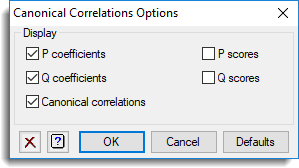1. Home
2. Canonical Correlations Analysis Options

# Canonical Correlations Analysis Options

This selects which results should be printed to the Output window by a canonical correlations analysis.## P coefficients and Q coefficients

These are the canonical variates or loadings, consisting of the coefficients of the linear combinations of P and Q determined by the analysis.

## Canonical correlations

These are the correlations between the vectors formed as corresponding linear combinations of P and Q. That is, the ith canonical correlation is the correlation between ui and vi, where ui and vi are the ith linear combinations of P and Q respectively, calculated using the ith canonical variates.

## P scores and q Scores

These are the scores or coordinates for the units obtained as linear combinations of the P and Q sets of variates.

Updated on April 26, 2019